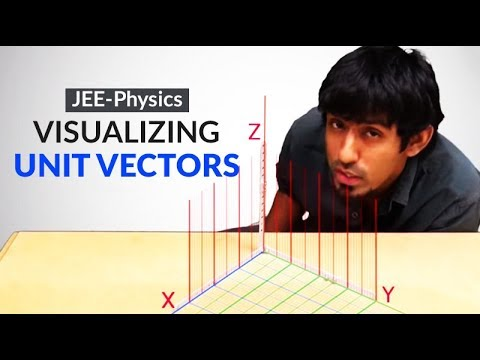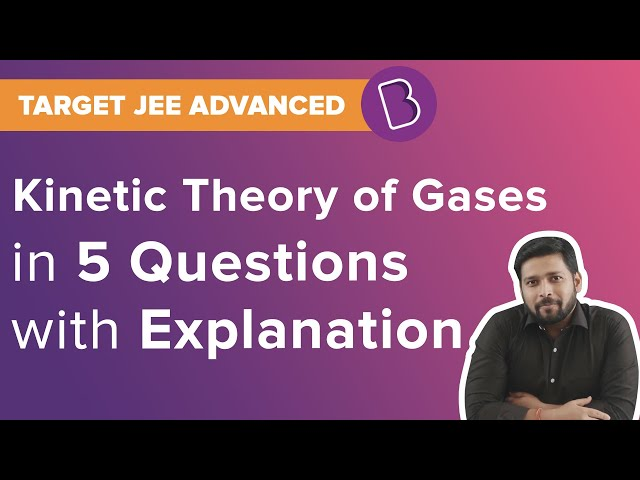Win up to 100% scholarship on Aakash BYJU'S JEE/NEET courses with ABNAT Win up to 100% scholarship on Aakash BYJU'S JEE/NEET courses with ABNAT

# JEE Advanced 2020 Physics Paper 2

Solved question paper of JEE Advanced 2020 Physics (Paper 2) is available on this page. Practising Physics Paper 2 will help the JEE aspirants to study productively and at the same time understand the coverage of Physics topics as well as the overall exam pattern. Solving previous years’ papers will help students to improve their speed and accuracy, which is one of the important factors to ace any of the exams. The questions and solutions can be either viewed on this page directly or PDF can be downloaded for offline practice.
Paper 1 – Physics

Question 1: A large square container with thin transparent vertical walls and filled with water (refractive index 4/3) is kept on a horizontal table. A student holds a thin straight wire vertically inside the water 12 cm from one of its corners, as shown schematically in the figure. Looking at the wire from this corner, another student sees two images of the wire, located symmetrically on each side of the line of sight as shown. The separation (in cm) between these images is ____________.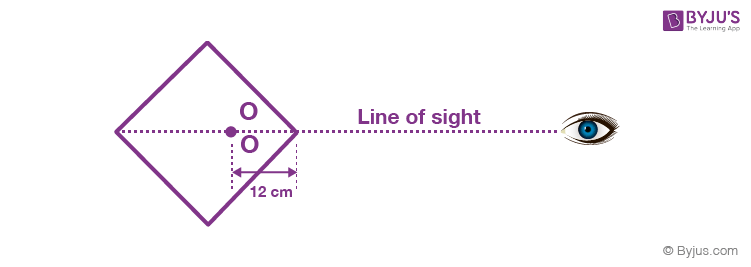For 2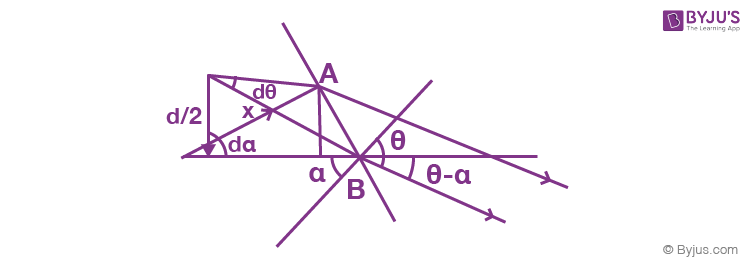We will assume that observer sees the image of object through edge. ⇒ α = 45°

AB = 12dα/cosα = xdθ/cosθ

By applying snell’s law,

(4/3) sin α = 1 sin θ

(4/3) cos α dα = cos θ dθ

9/(cos2 α) = x/(cos2 θ)

sin θ = sin α (4/3)

sin θ = (2√2)/3

x = 18/9 = 2

d = 2x sin(θ – α)

= 4 x 1/√2 [(2√2/3) – 1/3]

= 2 (approx)

For 3:

When ray is normally incident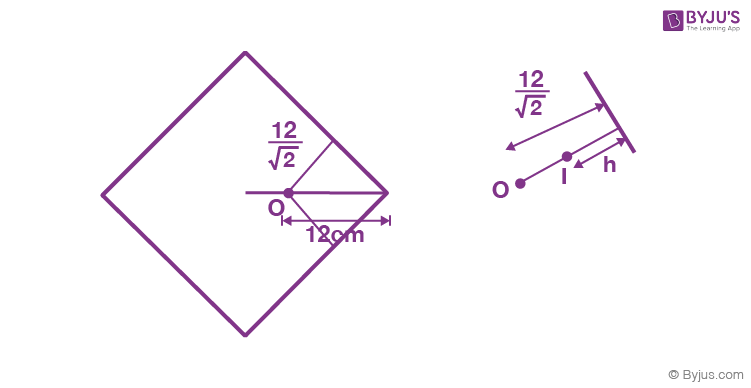h → apparent depth

h/(12/√2) = 1/(4/3)

or h = 9/√2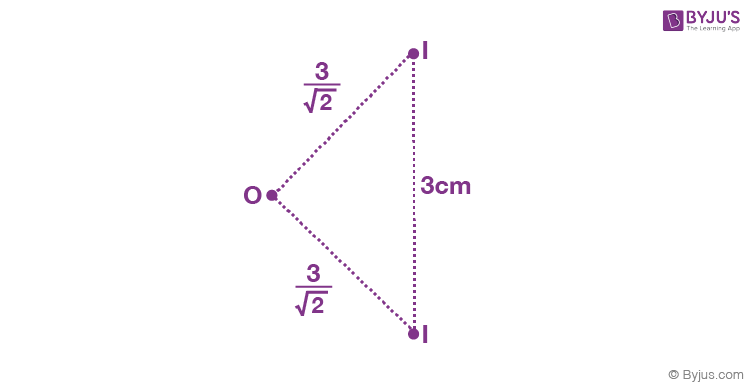So, distance between images will be 3 cm.

Question 2: A train with cross-sectional area 𝑆𝑡 is moving with speed 𝑣𝑡 inside a long tunnel of cross-sectional area 𝑆0 (𝑆0 = 4𝑆𝑡). Assume that almost all the air (density ρ) in front of the train flows back between its sides and the walls of the tunnel. Also, the airflow with respect to the train is steady and laminar. Take the ambient pressure and that inside the train to be 𝑝0. If the pressure in the region between the sides of the train and the tunnel walls is p, then p0 – p = (7/2N) ρ vt2. The value of N is ________.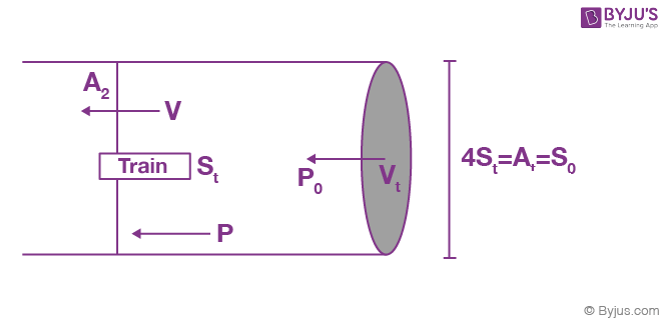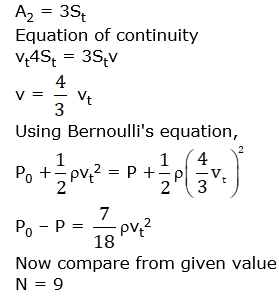Question 3: Two large circular discs separated by a distance of 0.01 m are connected to a battery via a switch as shown in the figure. Charged oil drops of density 900 kg m−3 are released through a tiny hole at the centre of the top disc. Once some oil drops achieve terminal velocity, the switch is closed to apply a voltage of 200 V across the discs. As a result, an oil drop of radius 8 × 10−7 m stops moving vertically and floats between the discs. The number of electrons present in this oil drop is ________. (neglect the buoyancy force, take acceleration due to gravity = 10 ms−2 and charge on an electron (e) = 1.6×10–19 C)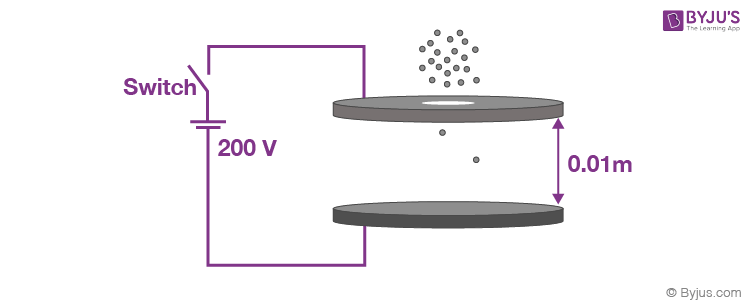qE = mg ….(i)

q = ne

V = Ed ⇒ E = V/d

from equation (i)

ne (v/d) = mg

n = mgd/eV

= 900 x 4π/3 x [8x8x8x10-21 x 10×0.01]/[1.6×10-19x200]

n = 6 (approx)

Question 4: A hot air balloon is carrying some passengers, and a few sandbags of mass 1 kg each so that its total mass is 480 kg. Its effective volume giving the balloon its buoyancy is V. The balloon is floating at an equilibrium height of 100 m. When N number of sandbags are thrown out, the balloon rises to a new equilibrium height close to 150 m with its volume V remaining unchanged. If the variation of the density of air with height h from the ground is ρ(h) = ρ0eh/h0, where ρ0 = 1.25 kg m-3 and ho = 6000 m, the value of N is ______.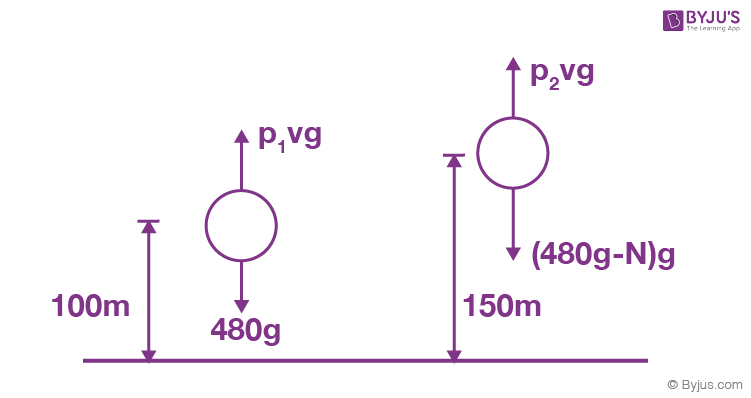Applying equilibrium,

ρ1vg = 480 g

ρ2vg = (480 – N) g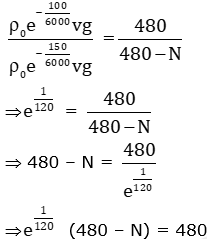N = 480(1 – e(-1/120)) = 480(1 – 0.9917) = 4 (approx.)

Question 5: A point charge q of mass m is suspended vertically by a string of length l. A point dipole of dipole moment vector p is now brought towards q from infinity so that the charge moves away. The final equilibrium position of the system including the direction of the dipole, the angles and distances is shown in the figure below. If the work done in bringing the dipole to this position is 𝑁 × (𝑚𝑔ℎ), where g is the acceleration due to gravity, then the value of N is _________ . (Note that for three coplanar forces keeping a point mass in equilibrium, F/sin θ is the same for all forces, where F is any one of the forces and θ is the angle between the other two forces)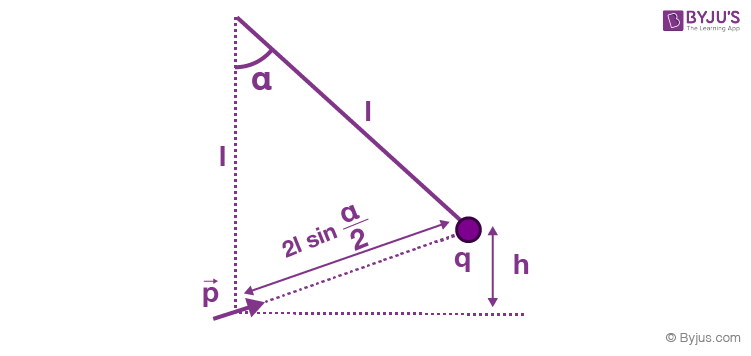Because α + 2 β = π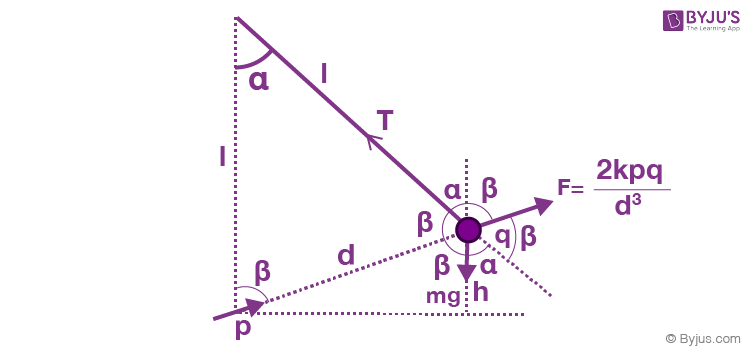β = (π/2 – α/2)

W = mgh + kpq/d2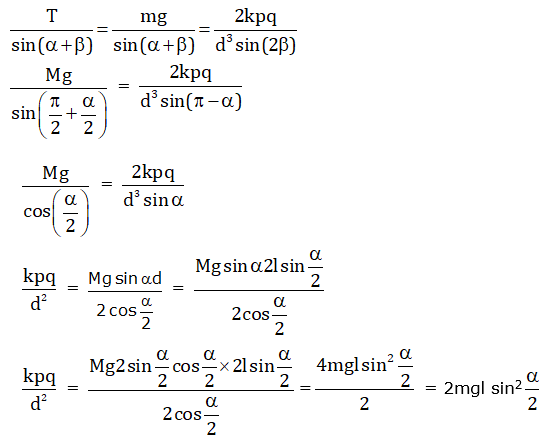Because cos β = h/d = h/2l sin(α/2)

Cos (π/2 – α/2) = h/2l sin(α/2)

Sin2(α/2) = h/2l

kpq/d2 = 2mgl x h/2I = mgh

w = mgh + kpq/d2 = mgh + mgh = 2mgh

N = 2

Question 6: A thermally isolated cylindrical closed vessel of height 8 m is kept vertically. It is divided into two equal parts by a diathermic (perfect thermal conductor) frictionless partition of mass 8.3 kg. Thus the partition is held initially at a distance of 4 m from the top, as shown in the schematic figure below. Each of the two parts of the vessel contains 0.1 mole of an ideal gas at temperature 300 K. The partition is now released and moves without any gas leaking from one part of the vessel to the other. When equilibrium is reached, the distance of the partition from the top (in m) will be _______ (take the acceleration due to gravity = 10 ms−2 and the universal gas constant = 8.3 J mol−1K−1).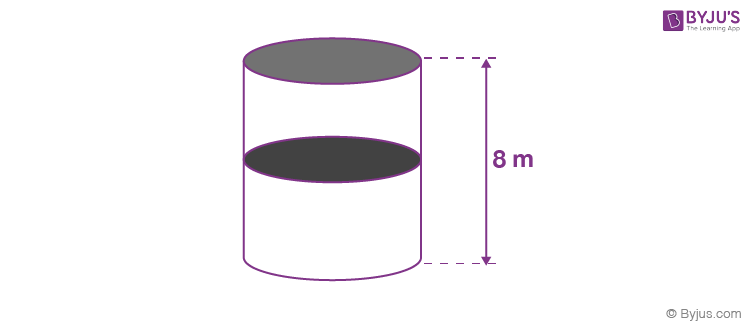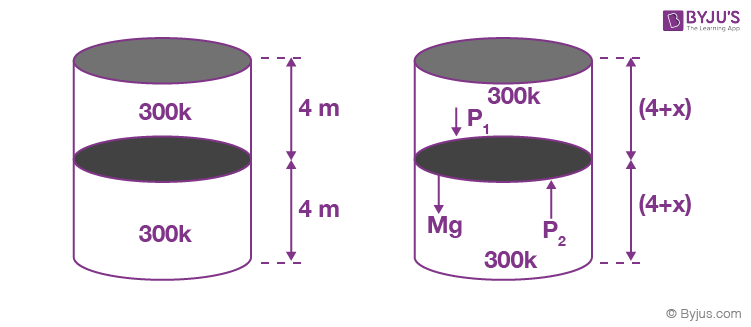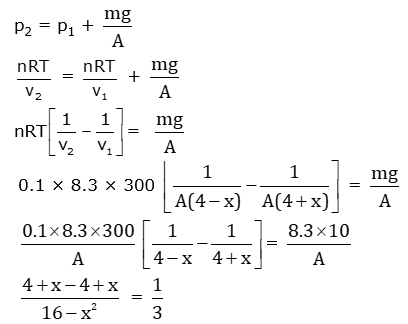6x = 16 – x2

x2 + 6x – 16 = 0

x = –8, 2

The distance of the partition from the top = 4 + 2 = 6 m

Question 7: A beaker of radius r is filled with water (refractive index 4/3) up to a height H as shown in the figure on the left. The beaker is kept on a horizontal table rotating with angular speed & omega;. This makes the water surface curved so that the difference in the height of water level at the centre and at the circumference of the beaker is h(h <<H, h << r), as shown in the figure on the right. Take this surface to be approximately spherical with a radius of curvature R. Which of the following is/are correct? (g is the acceleration due to gravity)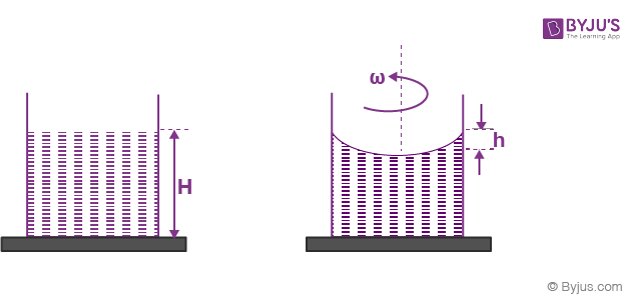a) R =

$$\begin{array}{l}\frac{h^2+r^2}{2h}\end{array}$$

b) R =
$$\begin{array}{l}\frac{3r^2}{2h}\end{array}$$

c) Apparent depth of the bottom of the beaker is close to

$$\begin{array}{l}\frac{3H}{2}(1+\frac{\omega^2H}{2g})^{-1}\end{array}$$

d) Apparent depth of the bottom of the beaker is close to

$$\begin{array}{l}\frac{3H}{4}(1+\frac{\omega^2H}{4g})^{-1}\end{array}$$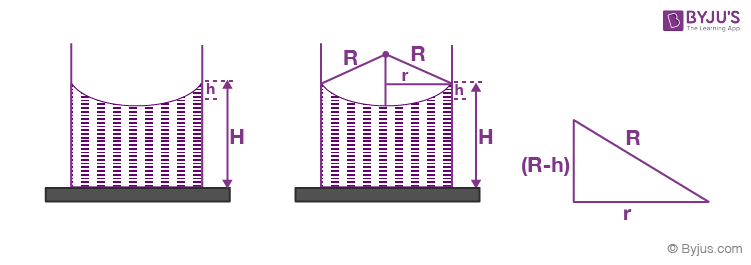By Pythagoras Theorem

R2 = (R – h)2 + r2

R2 = R2 + h2 – 2Rh + r2

2Rh = h2 + r2

R =

$$\begin{array}{l}\frac{h^2+r^2}{2h}\end{array}$$
…………. (1)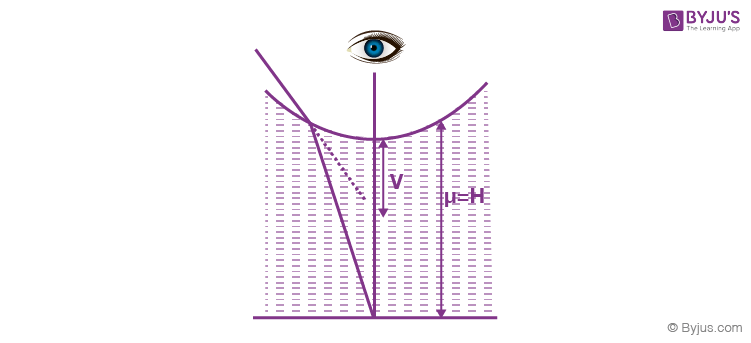The equation of refraction at a spherical surface is: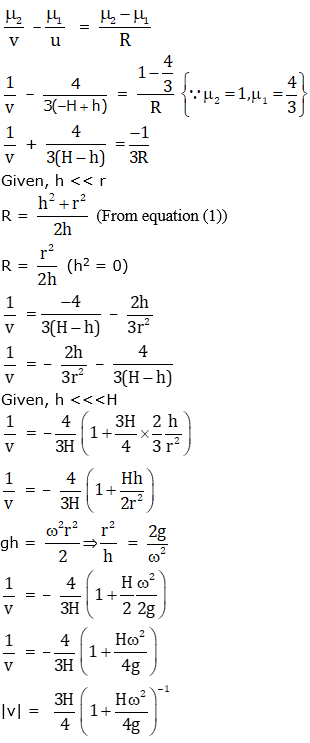Question 8: A student skates up a ramp that makes an angle 30° with the horizontal. He/she starts (as shown in the figure) at the bottom of the ramp with speed 𝑣0 and wants to turn around over a semi-circular path xyz of radius R during which he/she reaches a maximum height h (at point y) from the ground as shown in the figure. Assume that the energy loss is negligible and the force required for this turn at the highest point is provided by his/her weight only. Then (g is the acceleration due to gravity)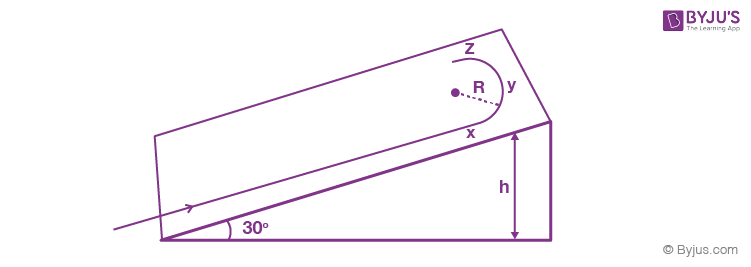a) v02 – 2gh = (1/2) gR
b) v02 – 2gh = (√3/2) gR
c) the centripetal force required at points x and z is zero
d) the centripetal force required is maximum at points x and z

Given: The force required for turn over a semi-circular path at the highest point is provided by his/her weight only so at that balancing situation

mg sin θ = mv2/R

mg sin 300 = mv2/R

gR/2 = v2 …(1)

From energy conservation

(1/2) mv02 = mgh + (1/2) mv2

v02 – 2gh = v2

v02 – 2gh = gR/2

Option a correct

For (d)

As gravitation force is not providing centripetal force at x and z that’s why maximum force is applied on x and z for circular motion.

Question 9: A rod of mass m and length L, pivoted at one of its ends, is hanging vertically. A bullet of the same mass moving at speed v strikes the rod horizontally at a distance x from its pivoted end and gets embedded in it. The combined system now rotates with angular speed ω about the pivot. The maximum angular speed ωM is achieved for x = xM. Then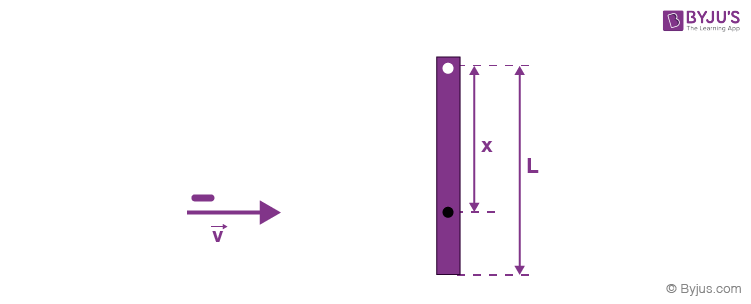a) ω =

$$\begin{array}{l}\frac{3vx}{L^2+3x^2}\end{array}$$

b) ω =
$$\begin{array}{l}\frac{12vx}{L^2+12x^2}\end{array}$$

c) xM = L/ √3
d) ωM = (v/2L) √3

From angular momentum conservation

Li = LF

mvx = (mL2/3 + mx2

m = mass of bullet and rod is same, v = speed of the bullet

ω = 3vx/(L2 + 3x2) …(i)

Option (A) is correct.

Now for maximum value of ω :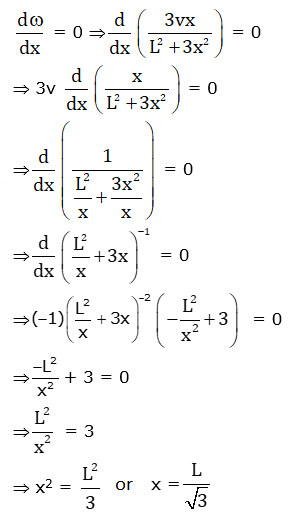Option (c) is correct

Now put this value in equation (i)

$$\begin{array}{l}\omega_{max} = \frac{3v(\frac{L}{\sqrt 3})}{L^2+3(L^2/3)} = \frac{\sqrt3 V}{2L}\end{array}$$

Option (D) is correct.

Question 10: In an X-ray tube, electrons emitted from a filament (cathode) carrying current I hit a target (anode) at a distance d from the cathode. The target is kept at a potential V higher than the cathode resulting in the emission of continuous and characteristic X-rays. If the filament current𝐼 is decreased to 1/2, the d potential difference Vis increased to 2v, and the separation distance d is reduced to d/2, then

a) the cut-off wavelength will reduce to half, and the wavelengths of the characteristic X-rays will remain the same
b) the cut-off wavelength, as well as the wavelengths of the characteristic X-rays, will remain the same
c) the cut-off wavelength will reduce to half, and the intensities of all the X-rays will decrease
d) the cut-off wavelength will become two times larger, and the intensity of all the X-rays will decrease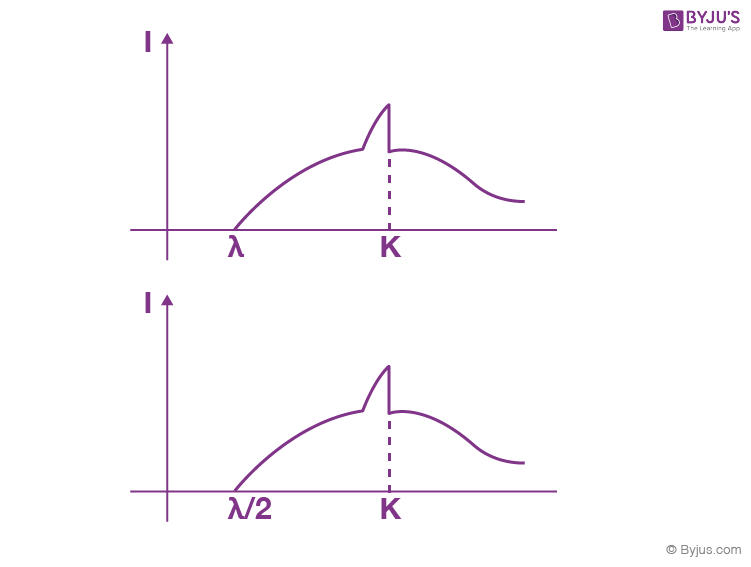As, E = qV

Here q = charge = e

&

E = hc/λ

eV = hc/λ

V ∝ 1/λ

or λ ∝ 1/v

V → 2V

λ → λ/2

If the current in the filament is reduced then emitting electrons should be reduced that’s why the intensity of x-ray is reduced.

Question 11: Two identical non-conducting solid spheres of same mass and charge are suspended in the air from a common point by two non-conducting, massless strings of the same length. At equilibrium, the angle between the strings is α. The spheres are now immersed in a dielectric liquid of density 800 kg m−3 and dielectric constant 21. If the angle between the strings remains the same after the immersion, then

a) electric force between the spheres remains unchanged
b) electric force between the spheres reduces
c) mass density of the spheres is 840 kg m−3
d) the tension in the strings holding the spheres remains unchanged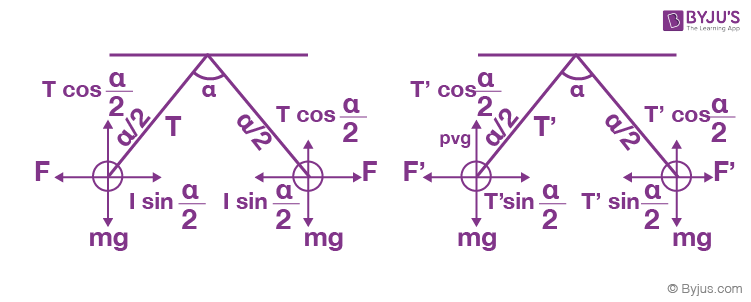Applying the equilibrium in a horizontal direction

T sin(α/2) = F

T’ sin(α/2) = F’

T/T’ = F/F’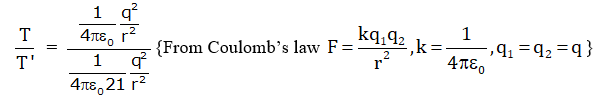T/T’ = 21/1

T’ = T/21

Applying the equilibrium in a vertical direction

T cos(α/2) = mg

T’ cos(α/2) = mg – ρ vg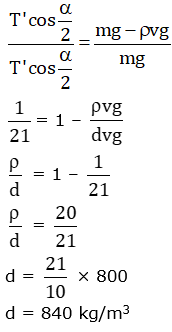Question 12: Starting at time 𝑡 = 0 from the origin with speed 1 ms−1, a particle follows a two-dimensional trajectory in the x-y plane so that its coordinates are related by the equation y = x2/2. The x and y components of its acceleration are denoted by 𝑎𝑥 and 𝑎y, respectively. Then

a) 𝑎𝑥 = 1 ms−2 implies that when the particle is at the origin, a𝑦 = 1 ms−2
b) 𝑎𝑥 = 0 implies 𝑎𝑦 = 1 ms−2 at all times
c) at 𝑡 = 0, the particle’s velocity points in the x-direction
d) 𝑎𝑥 = 0 implies that at 𝑡 = 1 s, the angle between the particle’s velocity and the x-axis is 45°

At t = 0, x = 0, u = 1 m/sec

y = x2/2

Differentiate with respect to t

Dy/dt = (2x/2) dx/dt

vy = x vx; vy = velocity component of y, vx = velocity component of x

ay = xax + vx2

Option a

dy/dx = x ; d2y/dx2 = 1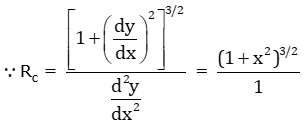at x = 0 Rc = 1

Therefore, ay = v2/Rc = 1 m/s2

at x = 0

ay = 1 m/s2

independent of ax

option (b)

if ax = 0

ay = 0 + vx2 = 1 m/sec2

option (c)

vy = xvx at x = 0 at t = 0

vy = 0 and vx = 1 m/sec

Option (d)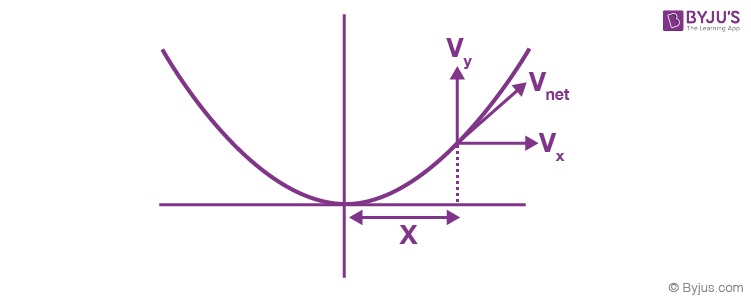x = 1m

vy / vx = tan θ = x = 1

[tan θ = (component of y)/ (component of x)]

So, θ = 45 degrees

Question 13: A spherical bubble inside water has radius R. Take the pressure inside the bubble and the water pressure to be p0. The bubble now gets compressed radially in an adiabatic manner so that its radius becomes (𝑅 − 𝑎). For 𝑎 << 𝑅 the magnitude of the work done in the process is given by (4 π𝑝0𝑅𝑎2)X, where X is a constant and γ = 𝐶𝑝⁄𝐶𝑉 = 41⁄30. The value of X is________.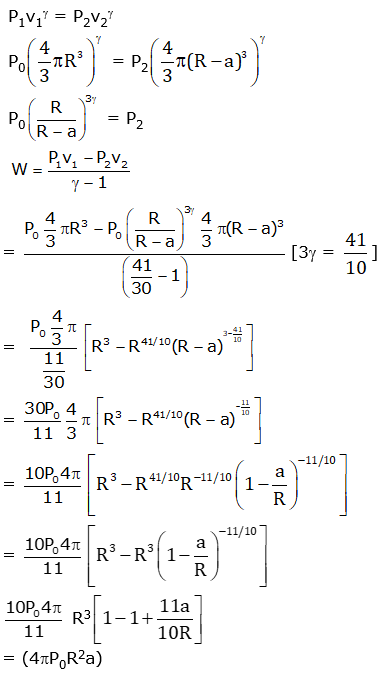Question 14: In the balanced condition, the values of the resistances of the four arms of a Wheatstone bridge are shown in the figure below. The resistance 𝑅3 has temperature coefficient 0.0004℃−1. If the temperature of 𝑅3 is increased by 100℃, the voltage developed between S and T will be __________ volt.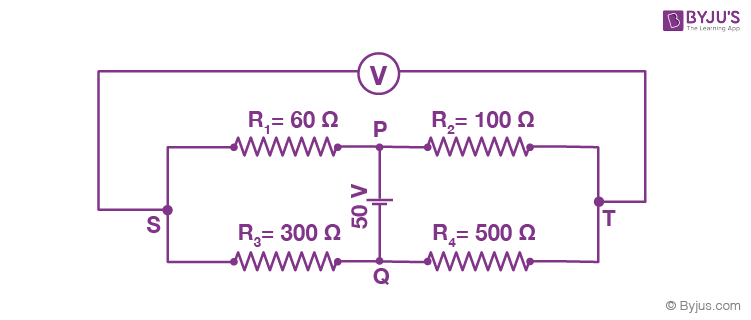R3 = 300 (1 + α ΔT)

R3 = 300 (1 + 0.0004 × 100)

R3 = 312 Ω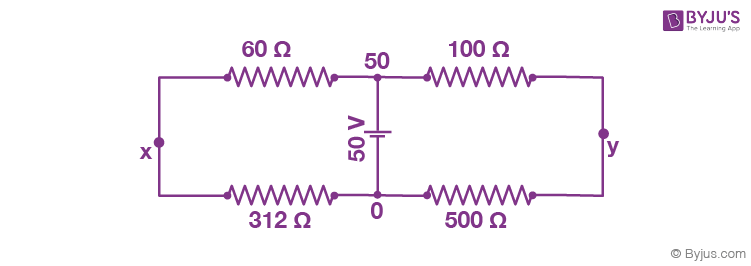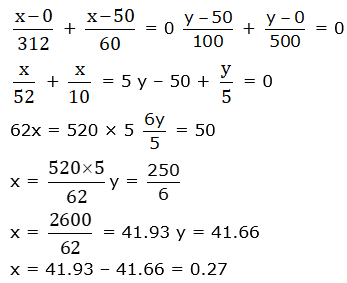Question 15: Two capacitors with capacitance values C1 = 2000 ± 10 pF and C2 = 3000 ± 15 pF are connected in series. The voltage applied across this combination is 𝑉 = 5.00 ± 0.02 V. The percentage error in the calculation of the energy stored in this combination of capacitors is _______.

ET = (1/2) Ceq V2

Assuming (Ceq = C)

1/C = 1/C1 + 1/C2

= 1/2000 + 1/3000

= 5/6000

C = 1200 pF

dF/E = dC/C + 2dV/V

1/C = 1/C1 + 1/C2

Finding dC/C: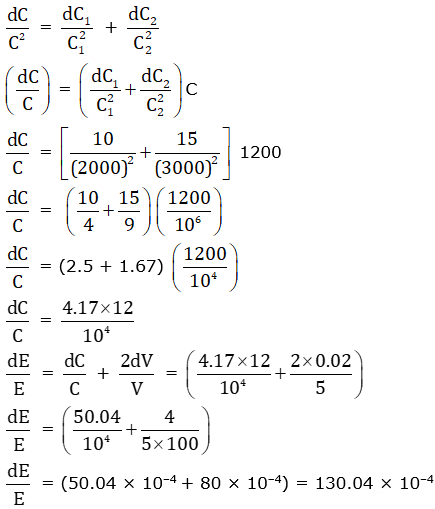Percentage Error:-

% dE/E = 130.04 x 10–4 x 100

= 1.30%

Question 16: A cubical solid aluminium (bulk modulus = -V (dP/dV) = 70 GPa) block has an edge length of 1m on the surface of the earth. It is kept on the floor of a 5 km deep ocean. Taking the average density of water and the acceleration due to gravity to be 103 kg m−3 and 10 ms−2, respectively, the change in the edge length of the block in mm is _____.

B = V (dP/dV)

Finding the magnitude of the change in the volume:-

70 x 109 = V/dV x 103 x 10 x 5 x 103

7 x 109 = V/dV x 106 x 5

7000 = V/dV x 5

dV/V = 5/7000

V = l3

dV/V = 3 dI/I

dI = 5/21000

dl = 0.238 mm = 0.24mm

Question 17: The inductors of two LR circuits are placed next to each other, as shown in the figure. The values of the self-inductance of the inductors, resistances, mutual-inductance and applied voltages are specified in the given circuit. After both the switches are closed simultaneously, then total work done by the batteries against the induced 𝐸𝑀𝐹 in the inductors by the time the currents reach their steady-state values is________mJ.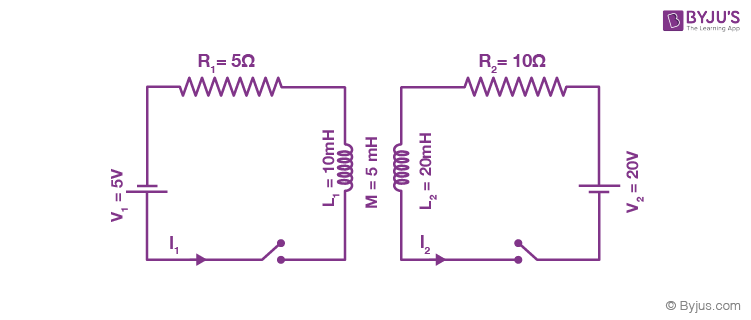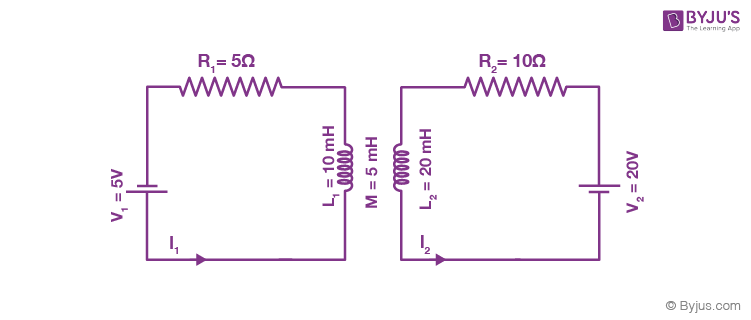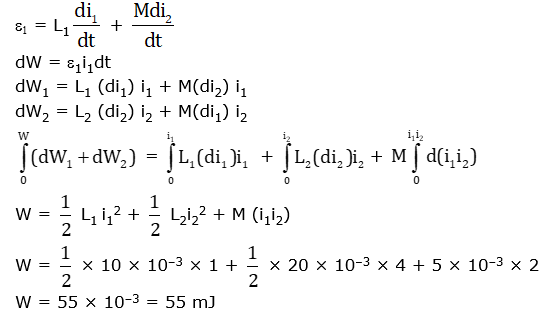Question 18: A container with 1 kg of water in it is kept in sunlight, which causes the water to get warmer than the surroundings. The average energy per unit time per unit area received due to the sunlight is 700 Wm−2 and it is absorbed by the water over an effective area of 0.05 m2. Assuming that the heat loss from the water to the surroundings is governed by Newton’s law of cooling, the difference (in ℃) in the temperature of water and the surroundings after a long time will be _____________. (Ignore the effect of the container, and take constant for Newton’s law of cooling = 0.001 s−1, the Heat capacity of water = 4200 J kg−1 K−1)

$$\begin{array}{l}\frac{dT}{dt} = (\frac{eA \sigma 4 T_o^3}{ms})(T-T_0)\end{array}$$

ms = 4200

$$\begin{array}{l}eA \sigma 4 T_o^3\end{array}$$
= 0.001 x 4200 = 4.2

Given, dQ/(dt x Area) = 700

dQ/dt = 700 x 0.05 = 35

$$\begin{array}{l}\frac{dQ}{dt} = (\frac{eA \sigma 4 T_o^3}{ms})(T-T_0)\end{array}$$

35 = 4.2 Δ T

Δ T = (35×10)/42 = 8.33

## Video Lessons – Paper 1 Physics